# Multi-plot grid in Seaborn

• Last Updated : 14 Jan, 2022

Prerequisites: Matplotlib, Seaborn

In this article, we are going to see multi-dimensional plot data, It is a useful approach to draw multiple instances of the same plot on different subsets of your dataset. It allows a viewer to quickly extract a large amount of information about a complex dataset. In Seaborn, we will plot multiple graphs in a single window in two ways. First with the help of Facetgrid() function and other by implicit with the help of matplotlib.

FacetGrid: FacetGrid is a general way of plotting grids based on a function. It helps in visualizing distribution of one variable as well as the relationship between multiple variables. Its object uses the dataframe as Input and the names of the variables that shape the column, row, or color dimensions of the grid, the syntax is given below:

Syntax: seaborn.FacetGrid( data, \*\*kwargs)

• data: Tidy dataframe where each column is a variable and each row is an observation.
• \*\*kwargs: It uses many arguments as input such as, i.e. row, col, hue, palette etc.

Below is the implementation of above method:

Import all Python libraries needed

## Python3

 `import` `seaborn as sns` `import` `numpy as np` `import` `pandas as pd` `import` `matplotlib.pyplot as plt`

Example 1: Here, we are Initializing the grid like this sets up the matplotlib figure and axes, but doesn’t draw anything on them, we are using the Exercise dataset which is well known dataset available as an inbuilt dataset in seaborn.
The basic usage of the class is very similar to FacetGrid. First you initialize the grid, then you pass plotting function to a map method and it will be called on each subplot.

## Python3

 `# loading of a dataframe from seaborn` `exercise ``=` `sns.load_dataset(``"exercise"``)`   `# Form a facetgrid using columns` `sea ``=` `sns.FacetGrid(exercise, col ``=` `"time"``)`

Output: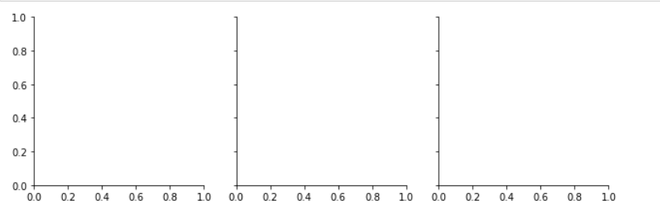Example 2: This function will draw the figure and annotate the axes. To make a relational plot, First, you initialize the grid, then you pass the plotting function to a map method and it will be called on each subplot.

## Python3

 `# Form a facetgrid using columns with a hue ` `sea ``=` `sns.FacetGrid(exercise, col ``=` `"time"``, hue ``=` `"kind"``)` `  `  `# map the above form facetgrid with some attributes   ` `sea.``map``(sns.scatterplot, ``"pulse"``, ``"time"``, alpha ``=` `.``8``)`   `# adding legend` `sea.add_legend()`

Output: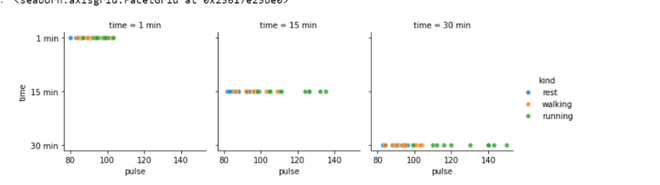Example 3: There are several options for controlling the look of the grid that can be passed to the class constructor.

## Python3

 `sea ``=` `sns.FacetGrid(exercise, row ``=` `"diet"``,` `                    ``col ``=` `"time"``, margin_titles ``=` `True``)`   `sea.``map``(sns.regplot, ``"id"``, ``"pulse"``, color ``=` `".3"``,` `        ``fit_reg ``=` `False``, x_jitter ``=` `.``1``)`

Output: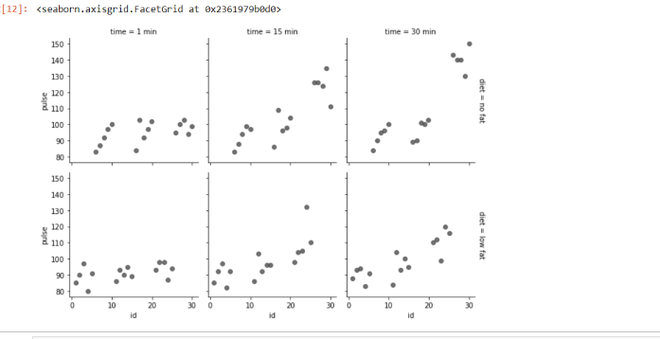Example 4: The size of the figure is set by providing the height of each facet, along with the aspect ratio:

## Python3

 `sea ``=` `sns.FacetGrid(exercise, col ``=` `"time"``,` `                    ``height ``=` `4``, aspect  ``=``.``5``)`   `sea.``map``(sns.barplot, ``"diet"``, ``"pulse"``,` `        ``order ``=` `[``"no fat"``, ``"low fat"``])`

Output: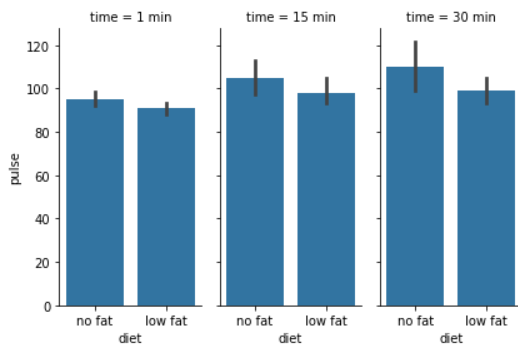Example 5: The default ordering of the facets is derived from the information in the DataFrame. If the variable used to define facets has a categorical type, then the order of the categories is used. Otherwise, the facets will be in the order of appearance of the category levels. It is possible, however, to specify an ordering of any facet dimension with the appropriate *_order parameter:

## Python3

 `exercise_kind ``=` `exercise.kind.value_counts().index` `sea ``=` `sns.FacetGrid(exercise, row ``=` `"kind"``, ` `                    ``row_order ``=` `exercise_kind,` `                    ``height ``=` `1.7``, aspect ``=` `4``)`   `sea.``map``(sns.kdeplot, ``"id"``)`

Output: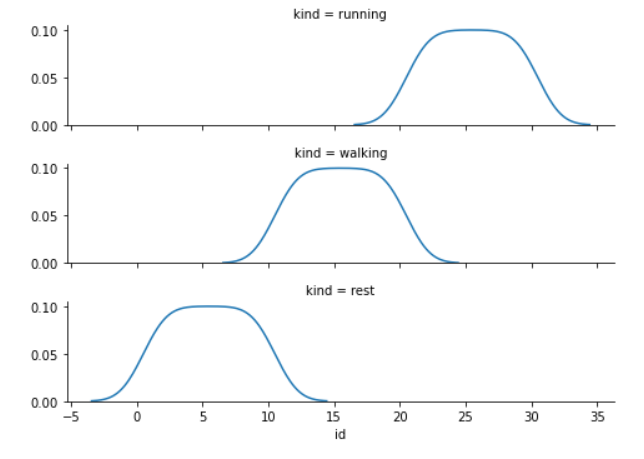Example 6: If you have many levels of one variable, you can plot it along the columns but “wrap” them so that they span multiple rows. When doing this, you cannot use a row variable.

## Python3

 `g ``=` `sns.PairGrid(exercise)` `g.map_diag(sns.histplot)` `g.map_offdiag(sns.scatterplot)`

Output: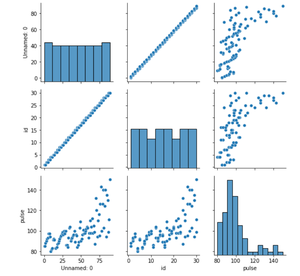Example 7:

In this example, we will see that we can also plot multiplot grid with the help of pairplot() function. This shows the relationship for (n, 2) combination of variable in a DataFrame as a matrix of plots and the diagonal plots are the univariate plots.

## Python3

 `# importing packages ` `import` `seaborn ` `import` `matplotlib.pyplot as plt `   `# loading dataset using seaborn ` `df ``=` `seaborn.load_dataset(``'tips'``) `   `# pairplot with hue sex ` `seaborn.pairplot(df, hue ``=``'size'``) ` `plt.show() `

Output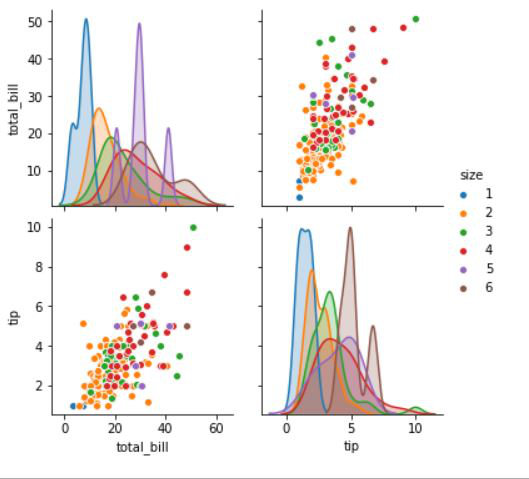Method 2: Implicit with the help of matplotlib.

In this we will learn how to create subplots using matplotlib and seaborn.

Import all Python libraries needed

## Python3

 `import` `seaborn as sns` `import` `numpy as np` `import` `pandas as pd` `import` `matplotlib.pyplot as plt`   `# Setting seaborn as default style even` `# if use only matplotlib` `sns.``set``()`

Example 1: Here, we are Initializing the grid without arguments returns a Figure and a single Axes, which we can unpack using the syntax bellow.

## Python3

 `figure, axes ``=` `plt.subplots()` `figure.suptitle(``'Geeksforgeeks - one axes with no data'``)`

Output: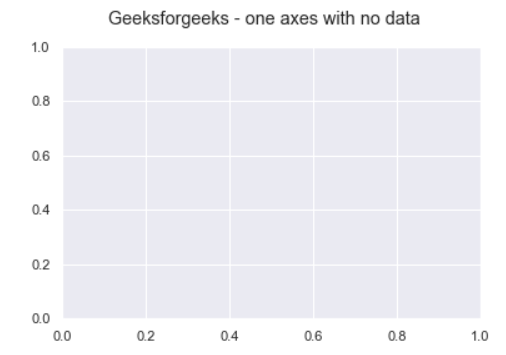Example 2: In this example we create a plot with 1 row and 2 columns, still no data passed i.e. nrows and ncols. If given in this order, we don’t need to type the arg names, just its values.

• figsize set the total dimension of our figure.
• sharex and sharey are used to share one or both axes between the charts.

## Python3

 `figure, axes ``=` `plt.subplots(``1``, ``2``, sharex``=``True``, figsize``=``(``10``,``5``))` `figure.suptitle(``'Geeksforgeeks'``)` `axes[``0``].set_title(``'first chart with no data'``)` `axes[``1``].set_title(``'second chart with no data'``)`

Output: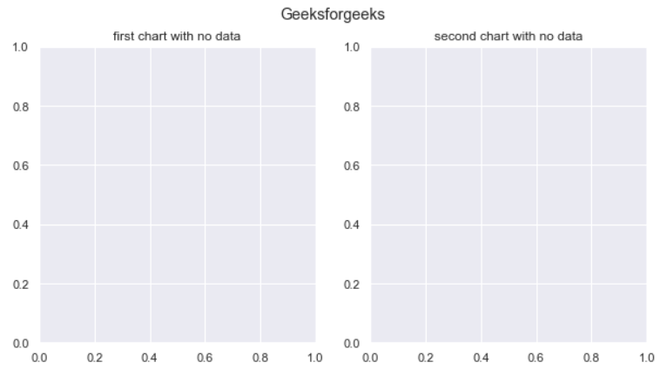Example 3: If you have many levels

## Python3

 `figure, axes ``=` `plt.subplots(``3``, ``4``, sharex``=``True``, figsize``=``(``16``,``8``))` `figure.suptitle(``'Geeksforgeeks - 3 x 4 axes with no data'``)`

Output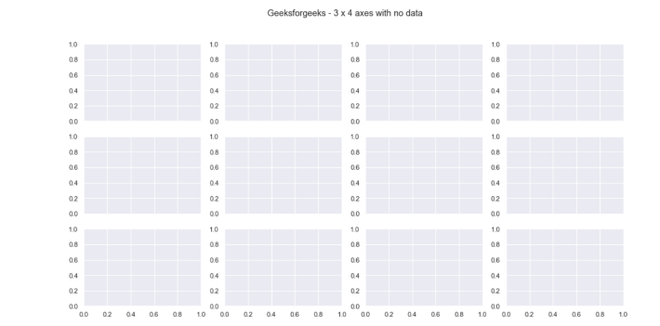Example 4: Here, we are Initializing matplotlib figure and axes, In this example, we are passing required data on them with the help of the Exercise dataset which is a well-known dataset available as an inbuilt dataset in seaborn. By using this method you can plot any number of the multi-plot grid and any style of the graph by implicit rows and columns with the help of matplotlib in seaborn. We are using sns.boxplot here, where we need to set the argument with the correspondent element from the axes variable.

## Python3

 `import` `seaborn as sns` `import` `numpy as np` `import` `pandas as pd` `import` `matplotlib.pyplot as plt`     `fig, axes ``=` `plt.subplots(``2``, ``3``, figsize``=``(``18``, ``10``))`   `fig.suptitle(``'Geeksforgeeks - 2 x 3 axes Box plot with data'``)`   `iris ``=` `sns.load_dataset(``"iris"``)`   `sns.boxplot(ax``=``axes[``0``, ``0``], data``=``iris, x``=``'species'``, y``=``'petal_width'``)` `sns.boxplot(ax``=``axes[``0``, ``1``], data``=``iris, x``=``'species'``, y``=``'petal_length'``)` `sns.boxplot(ax``=``axes[``0``, ``2``], data``=``iris, x``=``'species'``, y``=``'sepal_width'``)` `sns.boxplot(ax``=``axes[``1``, ``0``], data``=``iris, x``=``'species'``, y``=``'sepal_length'``)` `sns.boxplot(ax``=``axes[``1``, ``1``], data``=``iris, x``=``'species'``, y``=``'petal_width'``)` `sns.boxplot(ax``=``axes[``1``, ``2``], data``=``iris, x``=``'species'``, y``=``'petal_length'``)`

Output: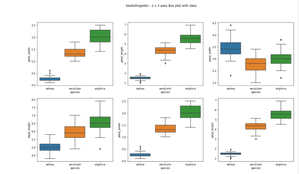Example 5: A gridspec() is for a grid of rows and columns with some specified width and height space. The plt.GridSpec object does not create a plot by itself but it is simply a convenient interface that is recognized by the subplot() command.

## Python3

 `import` `matplotlib.pyplot as plt`   `Grid_plot ``=` `plt.GridSpec(``2``, ``3``, wspace ``=` `0.8``,` `                         ``hspace ``=` `0.6``)`   `plt.subplot(Grid_plot[``0``, ``0``])` `plt.subplot(Grid_plot[``0``, ``1``:])` `plt.subplot(Grid_plot[``1``, :``2``])` `plt.subplot(Grid_plot[``1``, ``2``])`

Output: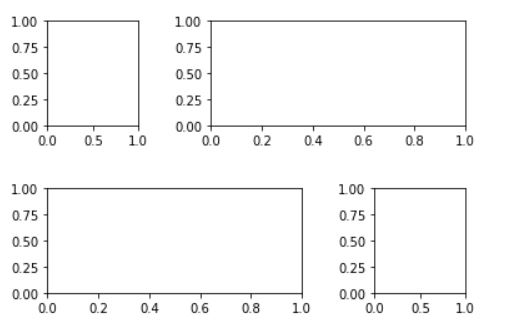Example 6: Here we’ll create a 3×4 grid of subplot using subplots(), where all axes in the same row share their y-axis scale, and all axes in the same column share their x-axis scale.

## Python3

 `import` `matplotlib.pyplot as plt`   `figure, axes ``=` `plt.subplots(``3``, ``4``,` `                            ``figsize ``=` `(``15``, ``10``))`   `figure.suptitle(``'Geeksforgeeks - 2 x 3 axes grid plot using subplots'``)`

Output: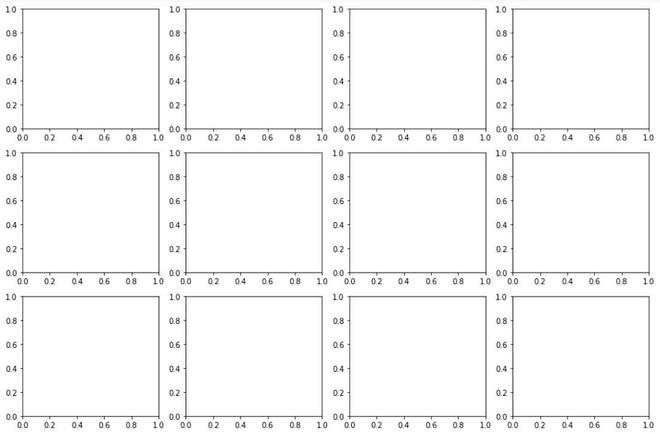My Personal Notes arrow_drop_up
Recommended Articles
Page :